# What is the logic behind the mirror symmetry method of solving circuits?

Frigus
I recently encountered mirror symmetry method of solving circuits and by it solving circuits became very easy but problem I am facing with it is that I can't figure out logic behind it.
For example if we try to simplify this circuit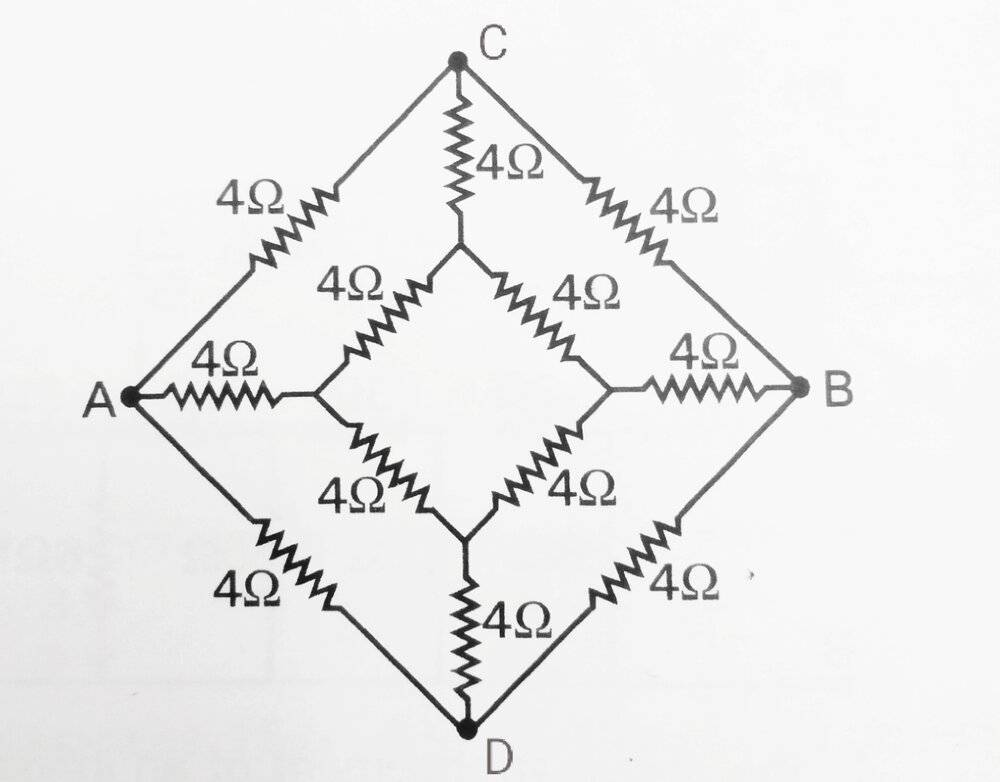Then we say that if ##I## current flows from Point A to C then equal current ##I## will flow from point A to D and after this if we reverse polarity of our battery then same current ##I## will flow from point B to C and same current ##I## flows from B to D and then from this we can conclude that if ##I## current flows from A to C then ##I## current should flow from point C to B and no current should flow from point C to D.
I am unable to understand what is the relation of this reasoning with the highlighted part,these both statements seems to have no relation with each other.
Thanks😃.

Let's say the voltage is applied between A and B. The current from A to C is ##i_{1}## and the current from C to B is ##i_{2}## (loosely, the currents are "toward the right"). If you reverse the voltage, all of the currents, in every branch, will change direction. The current from C to A is now ##i_{1}## and the current from B to C is now ##i_2## (loosely, the currents are now "toward the left").

Now imagine jumping into your computer and "looking at the screen from the other side" (alternatively, imagine the circuit is drawn on a window, and walk to the other side of the window). You will notice that this is exactly equivalent to what we had at the very start, except with the re-labelling ##A \rightarrow B## and ##B \rightarrow A##, and ##i_1## and ##i_2## have switched places. Hence why you can deduce ##i_1 = i_2##!

If you apply the same reasoning to a vertical branch in this example, you will get that ##i = -i##, or ##i = 0##!

Last edited by a moderator:
Staff Emeritus
It is very rare that we get to use symmetry in circuit analysis. Your illustration is a form of bridge circuit, which happens to be one of those rare cases.

You can approach it in more than one way. Kirchoff's Current Law (KCL) is one way. Consider point C, there are 3 branches at C, and KCL says that the sum of those currents must be zero. ##I_{AC}+I_{BC}+I_x=0## where the + direction of all three is into node C. But if ##I_{AC}+I_{BC}=0## by symmetry, then ##I_x=0## by KCL.

You can also see by symmetry that ##V_C=V_D=(V_A+V_B)/2##. And if ##V_C=V_D## then there should be no current flow between C and D.

Homework Helper
I recently encountered mirror symmetry method of solving circuits and by it solving circuits became very easy but problem I am facing with it is that I can't figure out logic behind it.
For example if we try to simplify this circuitView attachment 267200
Then we say that if ##I## current flows from Point A to C then equal current ##I## will flow from point A to D and after this if we reverse polarity of our battery then same current ##I## will flow from point B to C and same current ##I## flows from B to D and then from this we can conclude that if ##I## current flows from A to C then ##I## current should flow from point C to B and no current should flow from point C to D.
I am unable to understand what is the relation of this reasoning with the highlighted part,these both statements seems to have no relation with each other.
Thanks😃.
I see no battery in the circuit. However, it seems that the intent is that one is supposed to be connected at terminals A and B.

You seem to have no problem with the idea that current flowing on left (C side) and right (D side) should be the same. But you express doubt that the current flowing into from upstream (A side) should match current flowing out downstream (B side).

In the case of left and right it is just the same potential difference flowing across two identical arrangements of devices -- just mirror images of each other.

In the case of upstream and downstream it is similar. By symmetry, the potential at the dividing line has to be the average of the potential at the upstream and downstream terminals. The upstream and downstream arrangements are identical and are both subject to the same potential difference, so each carries the same current.

•Frigus
Frigus
Let's say the voltage is applied between A and B. The current from A to C is ##i_{1}## and the current from C to B is ##i_{2}## (loosely, the currents are "toward the right"). If you reverse the voltage, all of the currents, in every branch, will change direction. The current from C to A is now ##i_{1}## and the current from B to C is now ##i_2## (loosely, the currents are now "toward the left").

Now imagine jumping into your computer and "looking at the screen from the other side" (alternatively, imagine the circuit is drawn on a window, and walk to the other side of the window). You will notice that this is exactly equivalent to what we had at the very start, except with the re-labelling ##A \rightarrow B## and ##B \rightarrow A##, and ##i_1## and ##i_2## have switched places. Hence why you can deduce ##i_1 = i_2##!

If you apply the same reasoning to a vertical branch in this example, you will get that ##i = -i##, or ##i = 0##!
I have drawn this image as you had told to imagine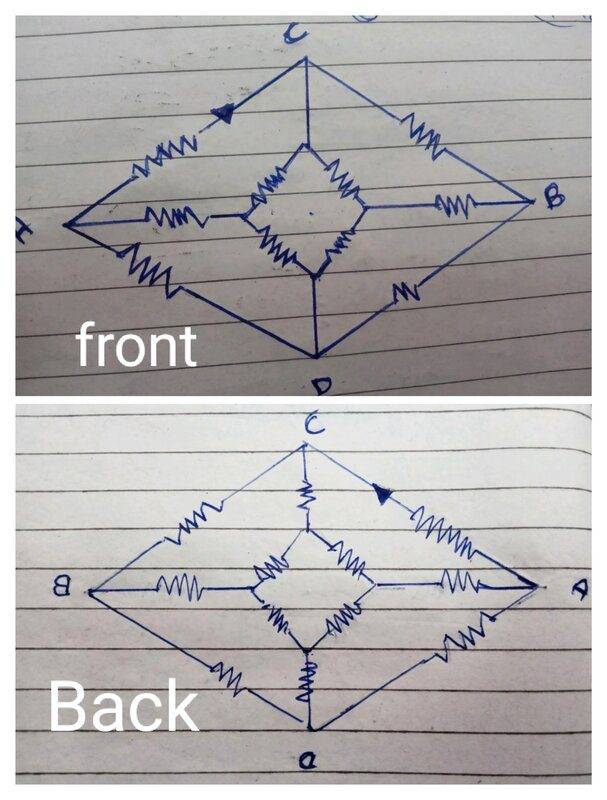But I am not able to deduce the conclusion (i.e ##I## entering the point C is equal to leaving the point C)
It seems to be almost like we reversed the battery polarity as you have told.
I can't understand why current entering the point C is equal to current leaving by using symmetry.
You can approach it in more than one way. Kirchoff's Current Law (KCL) is one way. Consider point C, there are 3 branches at C, and KCL says that the sum of those currents must be zero. IAC+IBC+Ix=0 where the + direction of all three is into node C. But if IAC+IBC=0 by symmetry, then Ix=0 by KCL.
It is reasonably understoodable from KCl that why current in CD is 0 but the thing which I am unable to understand is that why current entering is equal to leaving at point C.
But you express doubt that the current flowing into from upstream (A side) should match current flowing out downstream (B side).
Your method of firstly explaining question in clear words is very helpful as it prevents confusions that can erupt.
This is not the question I was asking,
I have tried to explain question again.
Hope it is clear now.

@Hemant I've annotated your original circuit with what I was getting at, hopefully it helps! Consider the original circuit, with node A at a potential of V volts and node B at 0 volts, with a the currents ##i_1## and ##i_2## as shown: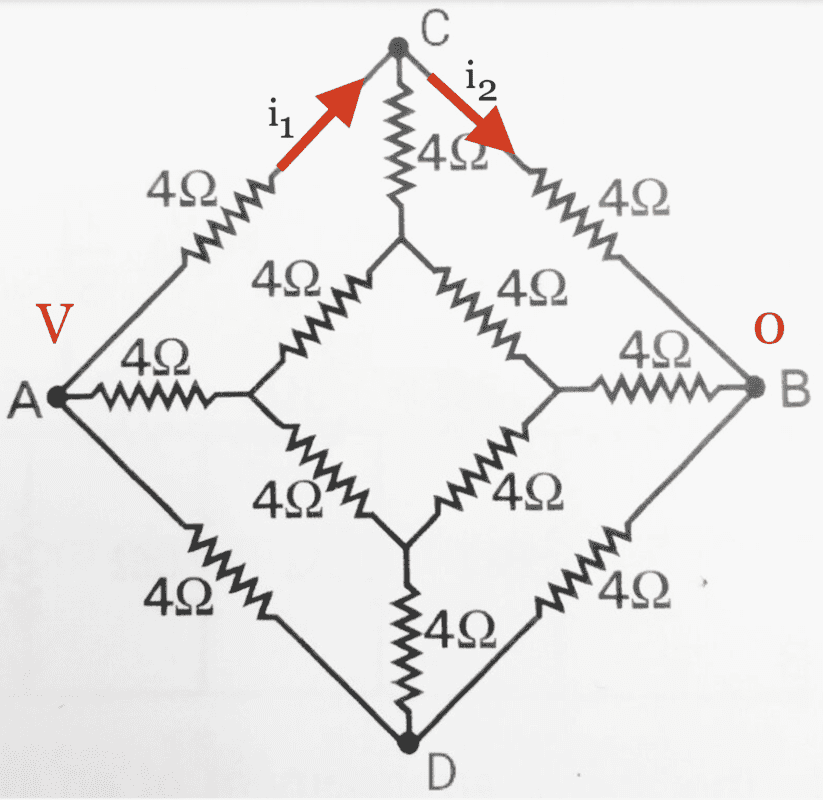Now, imagine reversing the voltage across the configuration (e.g. switching the leads from the battery), so all of the currents in the circuit change direction. You would end up with this: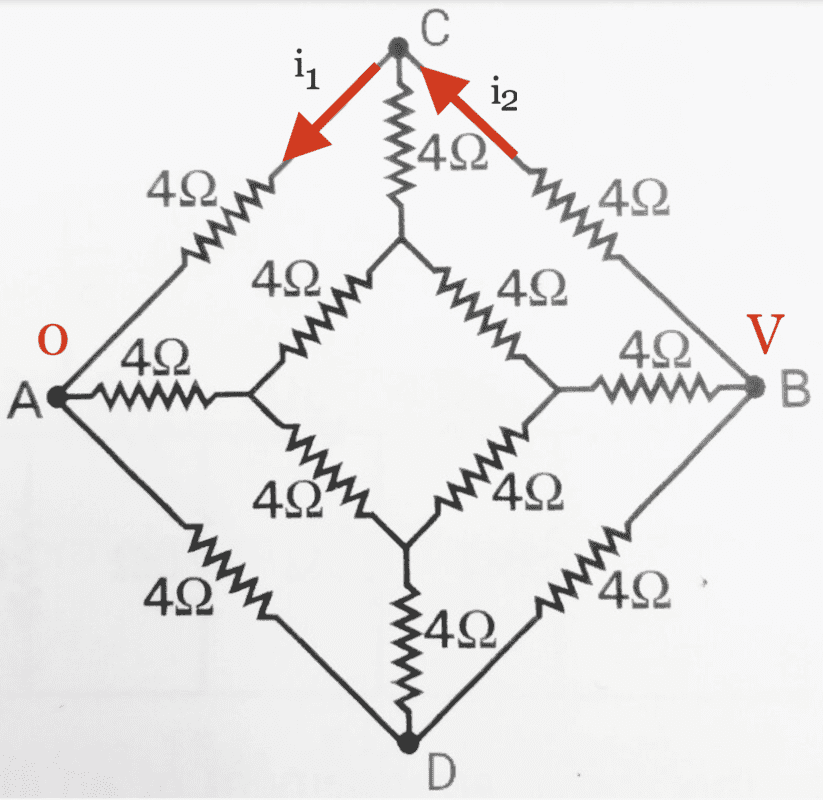But what does this look like when viewed from behind? It would look like this: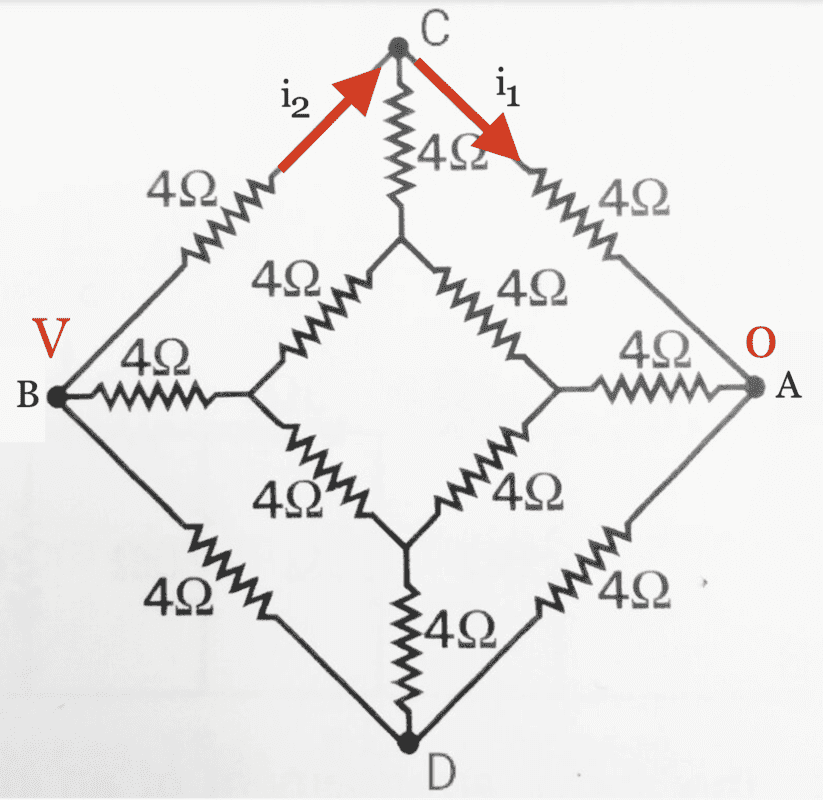But notice that this is exactly equivalent to our original circuit! To make it more obvious, let me put the first and third images side by side:Since what we've done is equivalent to a re-labelling of the circuit, hopefully you can see that ##i_1 = i_2##! There are a few other ways of thinking about this reflection symmetry, but I quite like this oneFrigus
@Hemant I've annotated your original circuit with what I was getting at, hopefully it helps! Consider the original circuit, with node A at a potential of V volts and node B at 0 volts, with a the currents i1and i2as shown:

View attachment 267206

Now, imagine reversing the voltage across the configuration (e.g. switching the leads from the l), so all of the currents in the circuit change direction. You would end up with this:

View attachment 267207

But what does this look like when viewed from behind? It would look like this:

View attachment 267208

But notice that this is exactly equivalent to our original circuit! To make it more obvious, let me put the first and third images side by side:

View attachment 267206View attachment 267208

Since what we've done is equivalent to a re-labelling of the circuit, hopefully you can see that i1=i2! There are a few other ways of thinking about this reflection symmetry, but I quite like this oneSorry for my bad explanation in recent post,
In my recent reply I wrote that I understand KCL but I don't understand why current entering at C is equal to current leaving the point C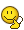.
If we take current I¹ as current entering the point A and moving towards point C when Point ##A## was connected to potential of ##V## and point ##B## to 0 volts of potential and now if we reverse the polarity of battery and say that current ##I²## enters at point ##B## and is moving towards point C then I can understand why current ##I¹## = ##I²## but the thing which I am unable to understand is that
If we connect point ##A## to voltage ##V## and ##B## to 0 then why all current entering the wire ##AC##(which is I¹) goes into Wire ##CB## without entering into ##CD##.
If we say that it is because at both ##C## and ##D## potential are
same then still there is some potential difference between point ##C## and ##E##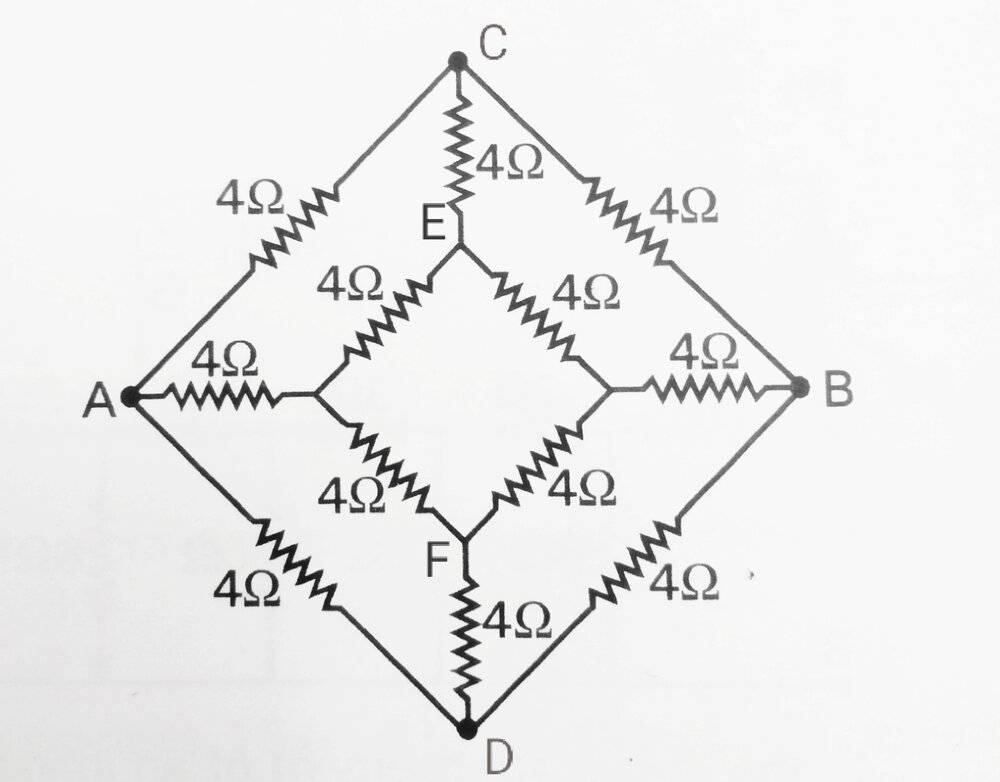So I think current should flow into Wire ##CE## as we can see that their is no symmetry of middle wire ##(AB)## with ##ACB## or ##ADB## wire.
I am sorry if question is still not clear.
Please tell me before answering the question that which part Is not clear.

If we take current I¹ as current entering the point A and moving towards point C

I suspect you mean the current leaving point A, toward point C, but this is just linguisticsYou are asking why the current in the branch CE is zero. First off, if you know the currents in AC and CB are equal from what we said above, then it follows directly from KCL that the current in CE is zero, as @anorlunda explained.

Alternatively, you could think of it like this. Suppose the current in branch CE is up, i.e. from E to C. Now reverse the voltage across the configuration, and all the currents will change direction; the current in branch CE will now be down, i.e. from C to E.

But it is mirror symmetry which tells you that, since the circuit is exactly the same when running from left to right as it is when running from right to left, the direction of the current in the branch CE must be the same in both cases! The only resolution is the current in CE is zero.

Last edited by a moderator:
Gold Member
Another two-cents worth.
Consider current to be a flow of Electrons (or water, for that matter!). If Electrons are entering a given point ('C' in your example) they either stay there (a capacitor) or leave, to the rest of the circuit.

But you may ask why they don't go to point E.

Well, consider a battery connected to A and B. In the outer circuit of ACB you notice that there is the same resistance between AC as there is between CB. Therefore the voltage on C is half the battery voltage.

You can use the identical logic on point E as used on point C. That is looking to the left (to point A) you have the same resistance as looking to the right (to point B).
Realizing this, you can see that the voltage on E is half the battery voltage.

With C and E being at half the battery voltage, there will be no current flowing between them.

Hope this helps.

Cheers,
Tom

p.s. I see etotheipi types faster than i do!•Frigus and etotheipi
Staff Emeritus
So I think current should flow into Wire CE a
Your confusion reminds me of another question we get here frequently on PF.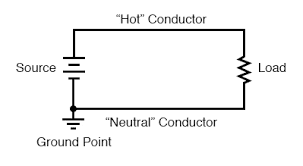Think of that circuit as a simplified power grid. The source is a power plant and the customer is the load. Confusion arises because the circuit is grounded. Students ask, "Why doesn't the current flow down into the ground?"

Consider that node just above the ground point. It is analogous to node C in your bridge.

The laws of circuit analysis say that for every amp that flows out of the top of that source, there must be exactly one amp that comes in from the bottom. The same amp flows through the load. If 1.00 amps flows from the node up to the source, and 1.00 amps come into the node from the load, how many amps flow into the ground? KCL tells us the answer; zero. Even though it is grounded, no current flows into the ground.

Apply the same logic to your bridge, you should see that current ##I_{CE}## must be zero because ##I_{AC}=I_{CB}##. There is no need to think about the other parts of the circuit between C and D, just focus on node C.

•Frigus, Motore and etotheipi
Frigus
Well, consider a battery connected to A and B. In the outer circuit of ACB you notice that there is the same resistance between AC as there is between CB. Therefore the voltage on C is half the battery voltage
I think I am missing some point🤔,
It makes sense to say that if only two resistance of same value are present in series then voltage drop across both the resistances should be equal so which means across each resistor voltage drop should be half of the battery but in this case in middle of resistors their present some another resistor maybe which can change the circuit like in this figure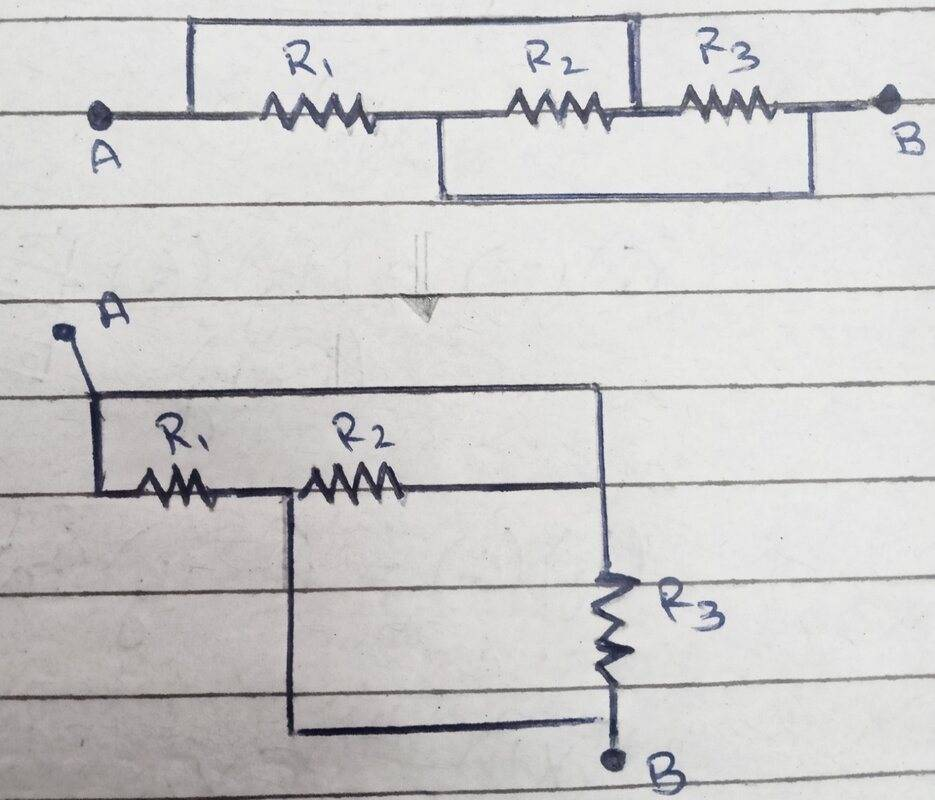the upper 3 resistors seems to be present in series at first sight (at least to me) but in reality case is different.
In nutshell I want to say that how do we knew that voltage drop across each resistor is same as we don't even know that if they are in series of not.
Alternatively, you could think of it like this. Suppose the current in branch CE is up, i.e. from E to C. Now reverse the voltage across the configuration, and all the currents will change direction; the current in branch CE will now be down, i.e. from C to E.
This gave me Eureka moment😃.
First off, if you know the currents in AC and CB are equal from what we said above, then it follows directly from KCL that the current in CE is zero, as @anorlunda explained.
I am not able to understand that why Current in AB and BC are equal,
Do we knew it by the case which gave me Eureka moment or is their some other logic present behind it.
Apply the same logic to your bridge, you should see that current ICE must be zero because IAC=ICB.
I can understand that by Kirchhoff current law that if all the current entering into node C moves towards the point B then current in CE should be 0 but the point which I am unable to understand is that
why ##I_{AC}=I_{AB}##?

I can understand that by Kirchhoff current law that if all the current entering into node C moves towards the point B then current in CE should be 0 but the point which I am unable to understand is that
why IAB##I_{AC}=I_{CB}## (corrected)?

That's the crux of the symmetry argument! The circuit has left-right mirror symmetry. There are a few different ways to think about it, e.g. by noting that the mirror line is an equipotential at ##V = \frac{V_A + V_B}{2}## like @anorlunda and @jbriggs444 described, or by thinking about how if you reverse the voltage you obtain an identical circuit (just flipped) like I went through.

Last edited by a moderator:
•Frigus
Staff Emeritus
There's another thing we didn't mention. If you know a branch carries zero current, you can remove it from the schematic. In your case, remove branches CE and DF.

Now what remains is two bridges in parallel between A and B. You can calculate the currents in each bridge separately because they don't interact with each other. In your case, forget the inner bridge. Now all you have left is nodes A B C D . Can you visualize the currents and symmetry in that? If so, you're all done.

How do you know they don't interact with each other? Think of two resistors in parallel connected to a battery V. Now I1=V/R1 and I2=V/2. I1 and I2 are not dependent on each other.

These symmetry arguments are unusual in circuit analysis. Beyond a simple Wheatstone Bridge, I don't know why teachers torture their students with examples like this one. I like to teach with circuits just complicated enough to show the concept, but not one bit more.

Frigus
by noting that the mirror line is an equipotential at V=VA+VB2 like @anorlunda and @jbriggs444 described
This makes sense to me,
If we consider some circuit like this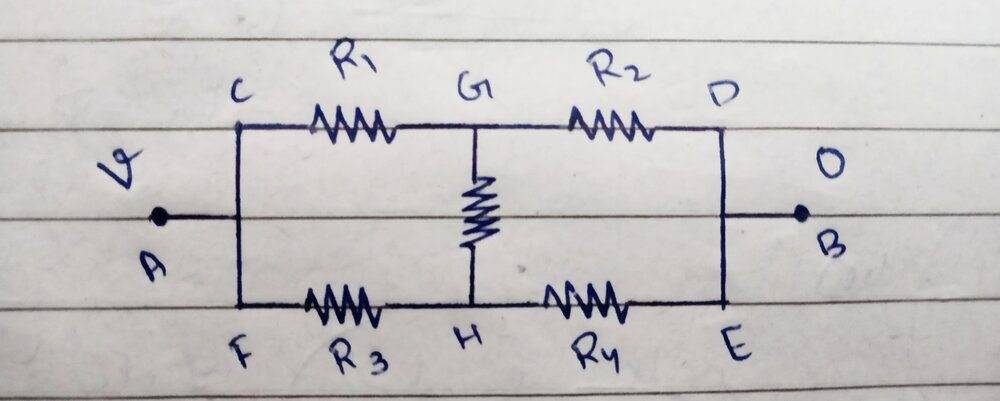Then it is clear that current in R₁ is equal to R₃(if they all are equal) thus ohm's law tells us that potential drop across R₁ and R₃ is same thus potential at points G and H is same which means no current in resistor between G and H,so it is very easy to see that why symmetry line is equipotential,
now problem is that I can't see that thing in the circuit I have posted,as resistor combination is different in line AB from ACB and ADB,
is there any general statement to prove that why potential at symmetry line is ##\frac {V_a +V_b}{2}## because it is not very satisfying to see for each circuit that why it is true.
Now all you have left is nodes A B C D . Can you visualize the currents and symmetry in that? If so, you're all done.
Yes,it is very easy to solve once we have removed resistors on symmetry line as after that it just became a good simple parallel circuit😁.
These symmetry arguments are unusual in circuit analysis. Beyond a simple Wheatstone Bridge, I don't know why teachers torture their students with examples like this one. I like to teach with circuits just complicated enough to show the concept, but not one bit more.
I am preparing for NEET exam in which we have to rely more on short tricks as we have to do 45 Physics question in 45 minutes and most of the questions have some point by which we can do it in 30-40 seconds like if their is circuit like this then if one remembered how to solve it using symmetry method then it very easy and if not then we have to apply kirchhoffs law and which requires at least 5 minutes which situation doesn't allows🙁.

is there any general statement to prove that why potential at symmetry line is ##\frac {V_a +V_b}{2}## because it is not very satisfying to see for each circuit that why it is true.

Put it this way... suppose for a minute that the voltage drops from A ##\rightarrow## C and C ##\rightarrow## B are different. Which one is greater, and which one is smaller?

Frigus
Put it this way... suppose for a minute that the voltage drops from A ##\rightarrow## C and C ##\rightarrow## B are different. Which one is greater, and which one is smaller?
if I try to move across path ACB then if current flows from point C to E then potential drop across resistor AC should be more than that of Resistor CB but if current flows from E to C then potential drop across Resistor CB is more than that of AC and if no current flows in CE then potential drop across each resistor should be same.You're not using the symmetry! The potential drops and currents across corresponding branches across the mirror line must be the same.

Staff Emeritus
Yes,it is very easy to solve once we have removed resistors on symmetry line as after that it just became a good simple parallel circuit😁.
is there any general statement to prove that why potential at symmetry line is Va+Vb2 because it is not very satisfying to see for each circuit that why it is true
Put those two statements together. After you removed the resistors and made is a simple parallel circuit, can you solve one of those parallel branches to show that ##V_C=\frac{V_A+V_B}{2}##?

•Frigus
Frigus
Put those two statements together. After you removed the resistors and made is a simple parallel circuit, can you solve one of those parallel branches to show that ##V_C=\frac{V_A+V_B}{2}##?
Yes,
Let's say E.M.F of battery=## V_a -V_b##
Considering wire ##ACB##,
There are two resistors of resistance ##R## which are in series,
So ##R_eq## = ##R_1+R_2##
##R_1=R ohm's##
##R_2=R ohm's ##
So
##R_eq## =R+R ohm's,
Now applying ohm's law,
##V## = ##I## ##R##
##I## = ##\frac {V}{R}##
##I## = ##\frac{V_a-V_b}{2R}##
So voltage drop across resistor ##R_1## should be
V= ##IR## = ##\frac{V_A-V_B}{2R}## ##R## and we know that potential at point A is ##V_a##
So potential at point c should be ##V_a - IR_1##
Now after putting the values we get,
= ##V_a## - ##\frac{V_A-V_B}{2R}## ##R## => ##V_C=\frac{V_A+V_B}{2}##
Hence proved😁.
I am really Thankfull to all for clearing my doubt.

Last edited:
•Tom.G and anorlunda